# The Formula Column - Explanation and Use Cases

Missing the Excel formula and mathematic capabilities? Not to worry! We came up with the formula column just for this. This column allows you to make calculations with other columns. It goes from simple mathematical equation to more complex functions and formulas.Tip: You can watch our recorded formula webinar right here :)

To add it, click on the + icon located on the top right of your board and select "column center".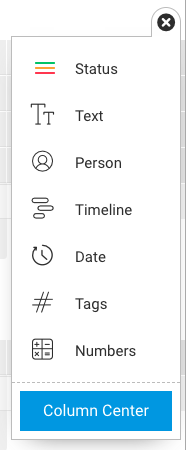Once in the center, select the option "Formula" as in the screenshot below: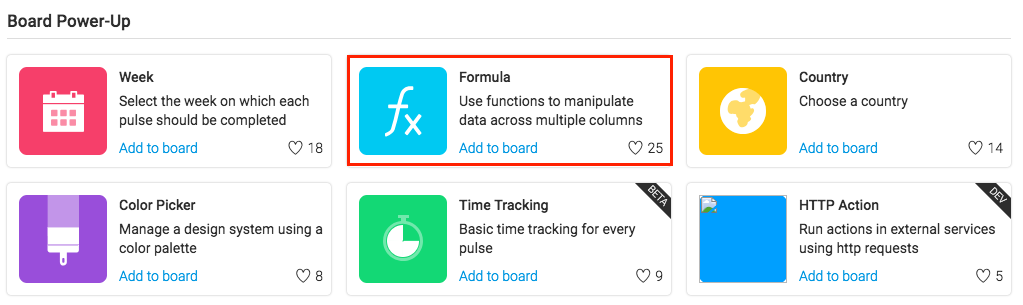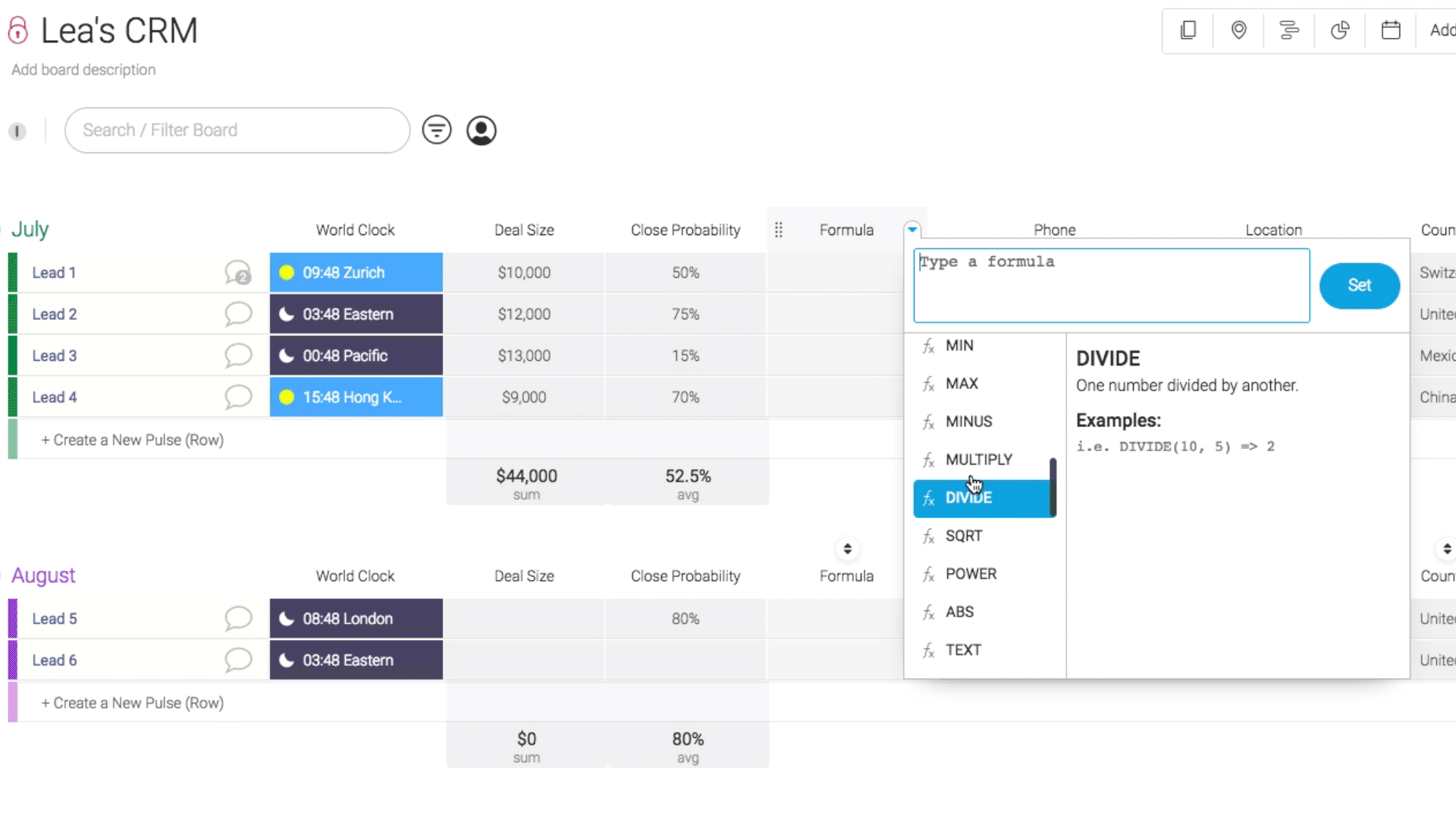Note: The formula column is only available for the Pro and Enterprise plan. If you were on a Pro plan and downgraded your account, the formula columns you have created will remain intact, but you will not be able to add more formula columns.

# Can I add a unit for my formula?

Yes you can 😊. You can add any unit before or after your formula. Here is how it works:Tip: The quotes around your unit are super important and a part of the formula. Don't forget them!

# Can I format my formula?

You can format your result exactly the way you want it to look like by using the formula TEXT. Let's take an easy example: I have want to multiply 10 by 1250 and I want my number to show the following way: \$12,500.00.Formula: TEXT(MULTIPLY({Deals},{Amount}),"\$#,##.00")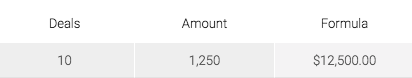Each # represents a number. I have added .00 by the end because I want my formula to end by .00 but you can replace this by .## if you want your number to end with proper numbers.

# Can I add a sum at the bottom of my formula column?

Yes you can! Exactly like for the numbers column, you can see the overall calculation for each group.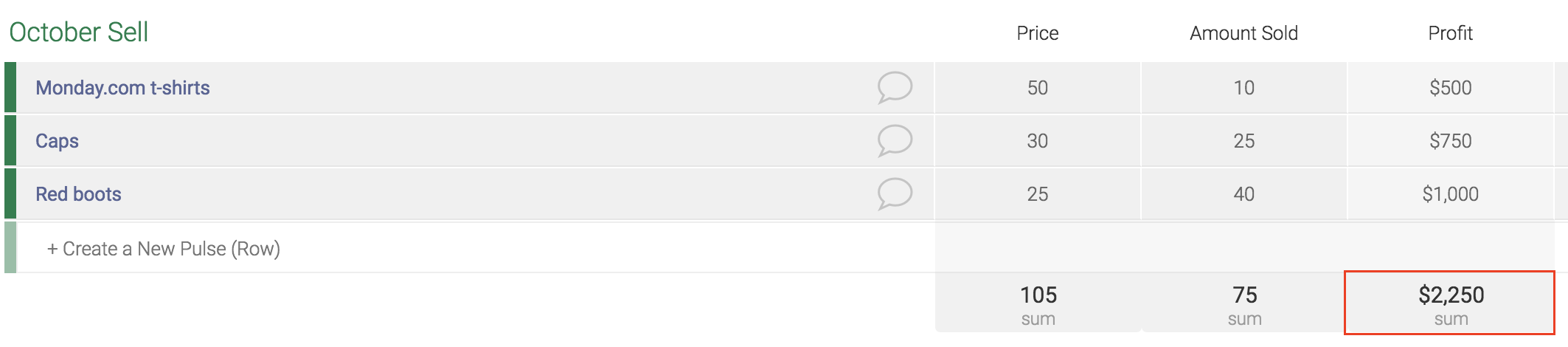By clicking on this box, you will also be able to change the unit and the function as below: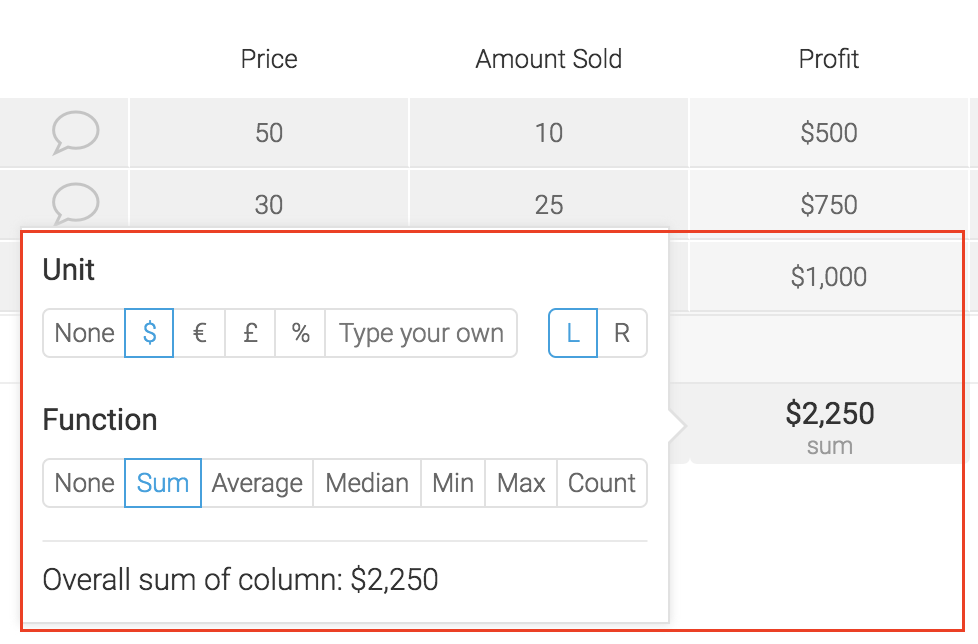Note: The total only takes into account numeric values. If your column has both numeric and text values, we will only count the numeric values. Mixed values like Lea123 will not be taken into account in the total. If all values are non-numeric, you will get N/A at the bottom as in the screenshot below.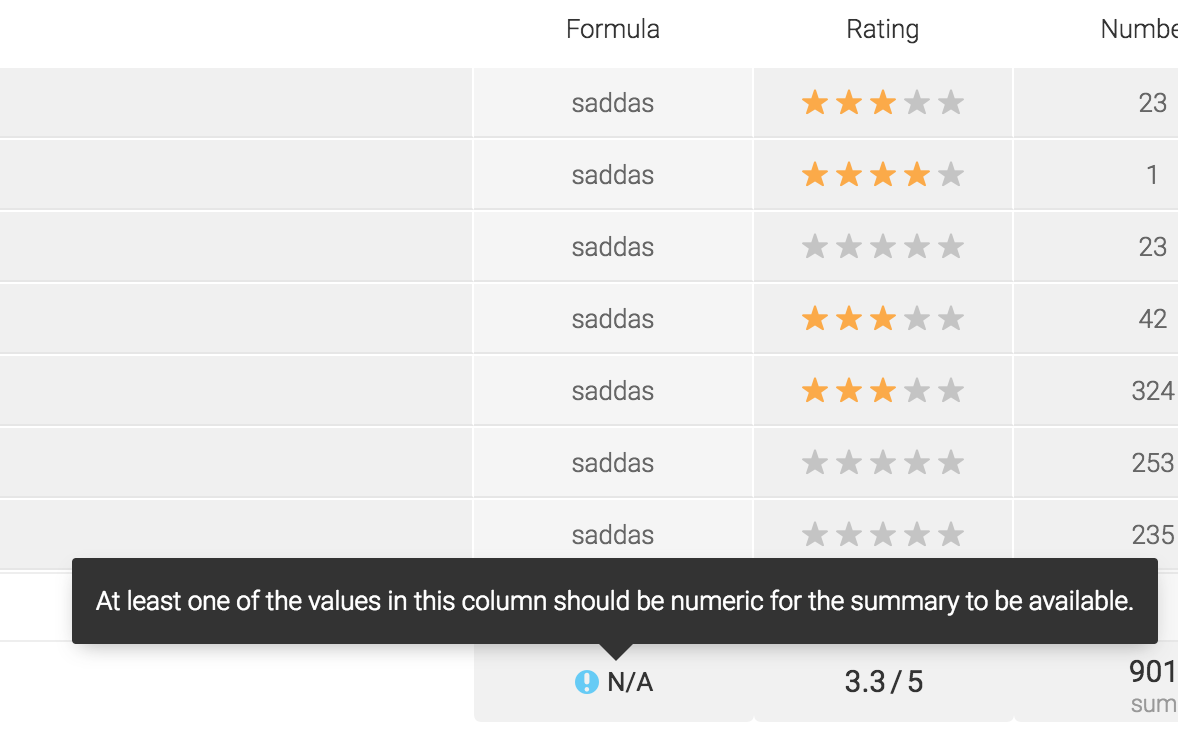# Use Cases for sales teams

Calculate the monthly commissions of each sales representative

In this example, I want to calculate the total amount of commission made per employee per month in £.Formula: ({Deals}*({%Commission}/100))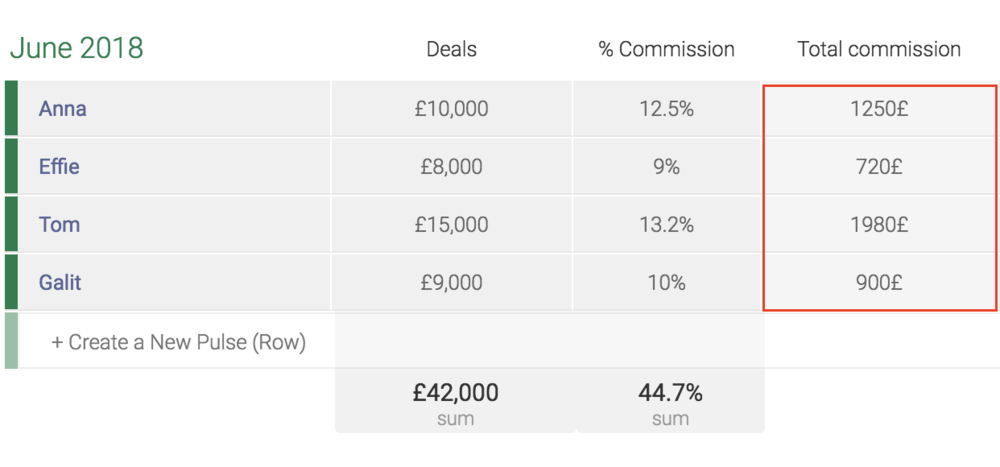Calculate the monthly commissions with conditions

Calculate if your employees are entitled to get a bonus. An employee can get a bonus only IF the "total sales" is higher than \$350,000 AND IF the amount of deals is higher than 12.Formula: IF(AND({Total Sales}>350000,{Deals}>12),"\$250","\$0")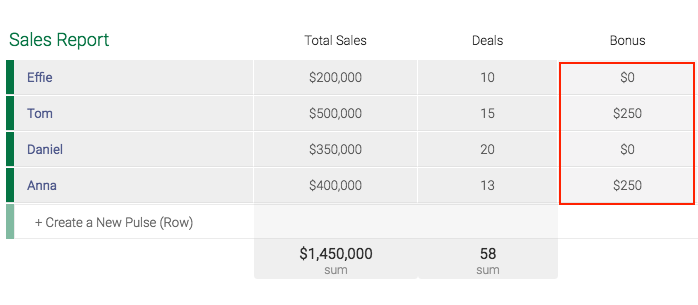Calculate the monthly change % between two values

I want to calculate the monthly change between the results of January and February.Formula: (MINUS({February Total Sales},{January Total Sales}))/{January Total Sales}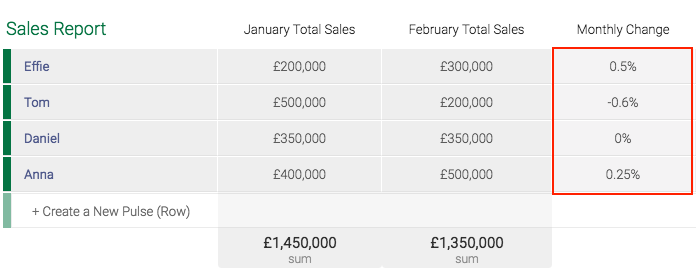# Use Cases for budget planning

Calculate the roll-over budget

Calculate the rollover budget (planned cost vs. actual cost) to see how much money you have left for a project.Formula: (MINUS({Planned Cost},{Actual Cost})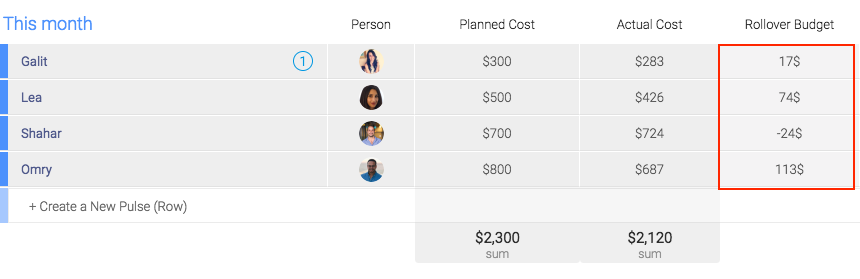Calculate the total expenses of a project

We are sending some of our employees to our NYC office and have a few expenses to take into consideration: the flights, the insurance, the hotel and the food expenses.Formula: SUM({Flight},{Hotel},{Insurance},{Expenses})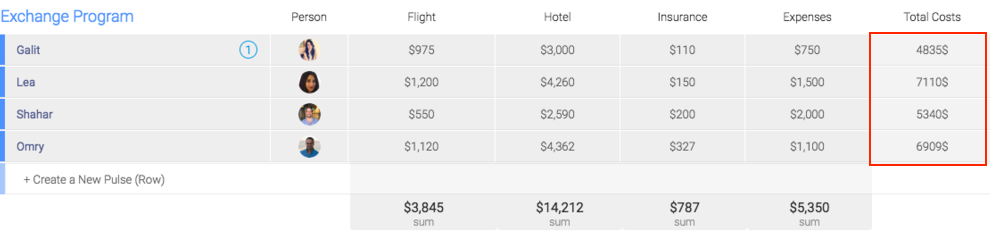Find if your total expenses are over budget or in budget

My budget is \$6500 per employee. I want to find out if the total amount I spent for each employee is in the budget or over it.Formula: IF((SUM({Flight},{Hotel},{Insurance},{Expenses}))>6500, "Over Budget","Good")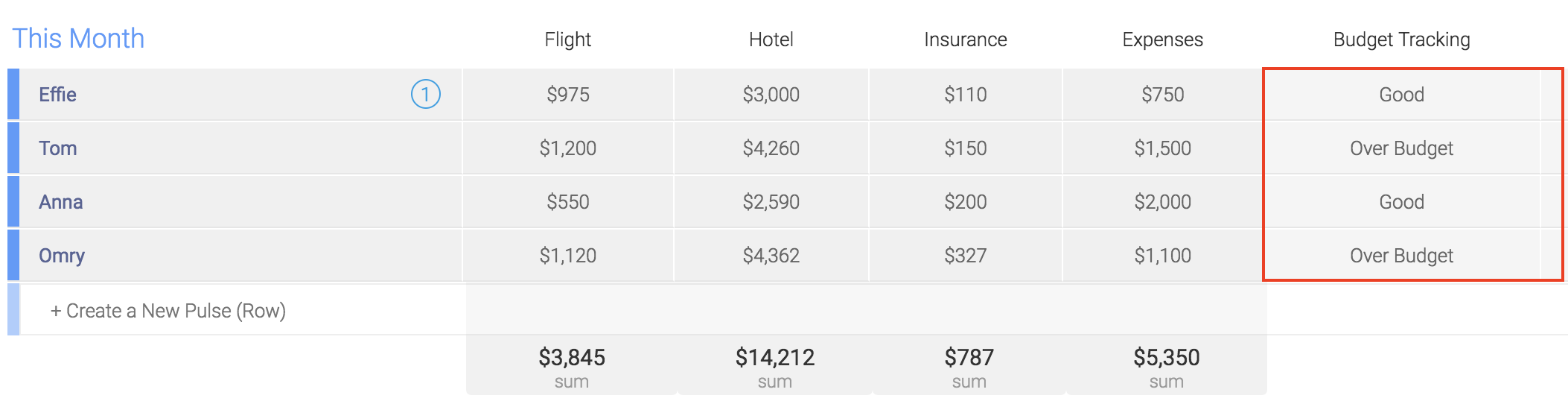Cost calculation of a project

I want to calculate the cost to perform a task based on the fee per hour and the total amount of hoursFormula: MULTIPLY({Number of hours},{Rate per hour})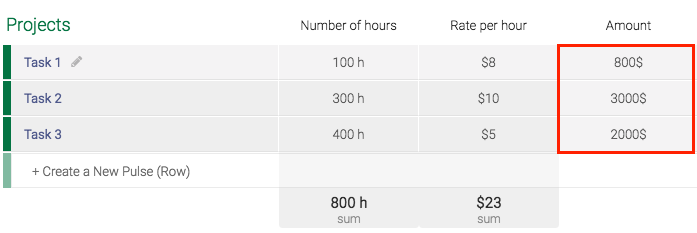Calculate the profit margin

To calculate the profit margin of each of your project, you need three data: the revenue, the cost and the profit. From there, you apply the following formula:Formula: ROUND({Profit}/{Revenue}*100, 1)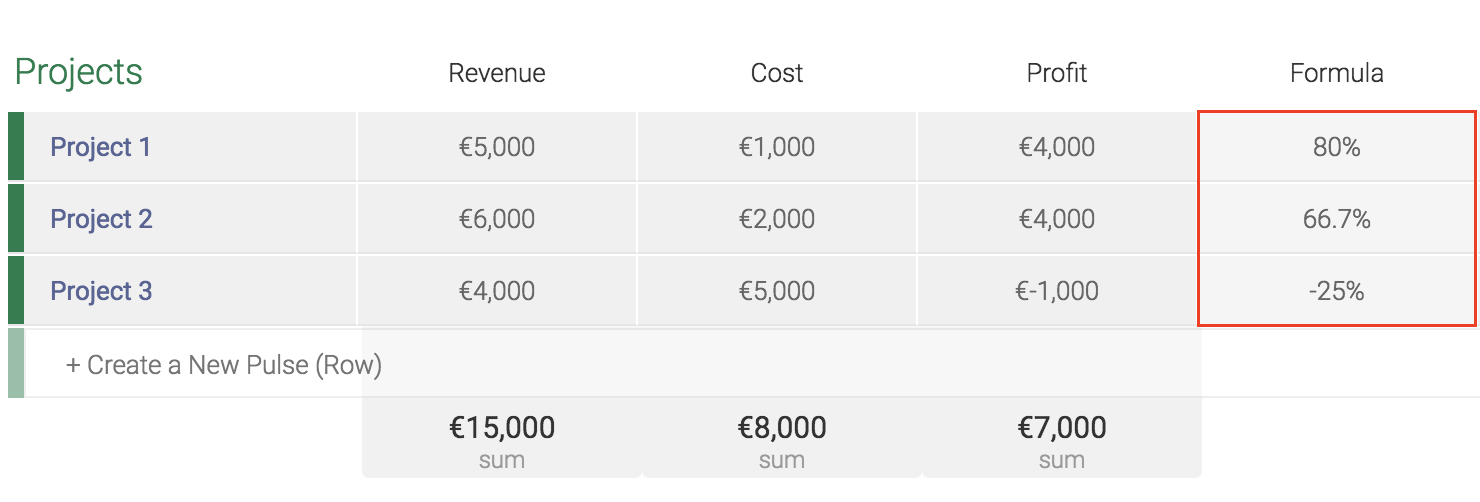Calculating the billing rate based on the time tracking columnFormula: ROUND(MULTIPLY(({Billable}/3600),{Hourly Rate}),1)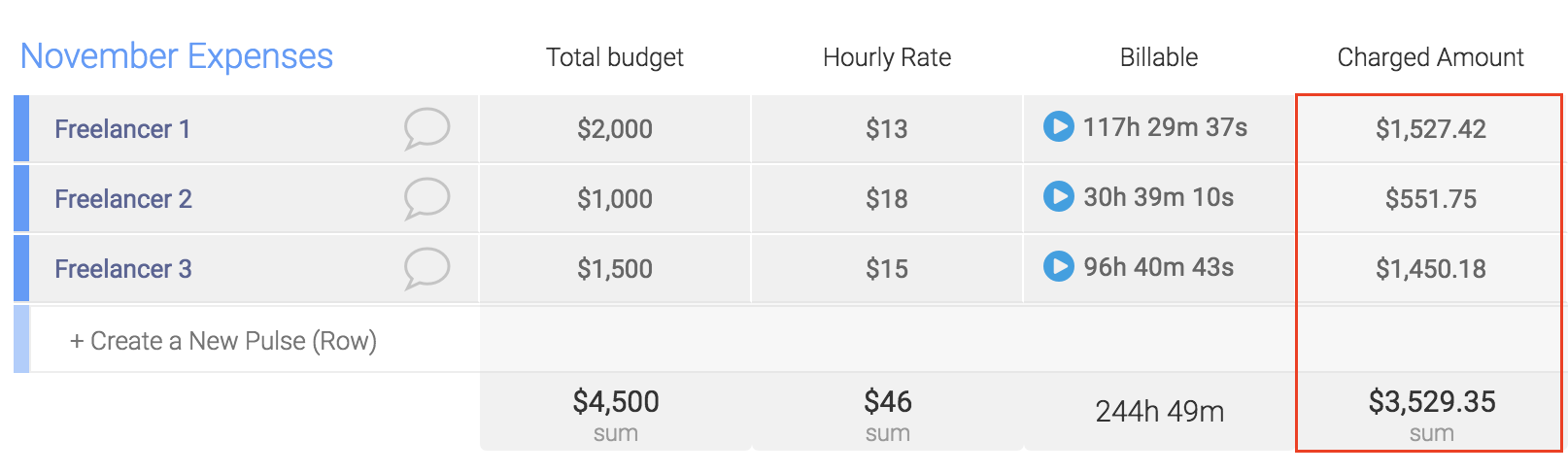Calculate the average between several number columns

In this example, we want to calculate the average of the grades for the last 4 weeksFormula: AVERAGE({Week 1},{Week 2},{Week 3},{Week 4})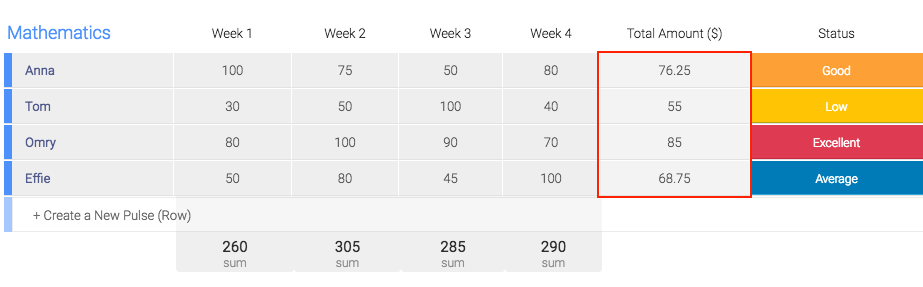# Use Cases for inventory

You can easily manage your inventory with monday.com. This example explains you how to calculate your current available stock and how much were sold in percentage.Formula: MINUS({Purchased},{Reserved},{Sold})Formula: ROUND({Sold}/{Purchased}*100,2)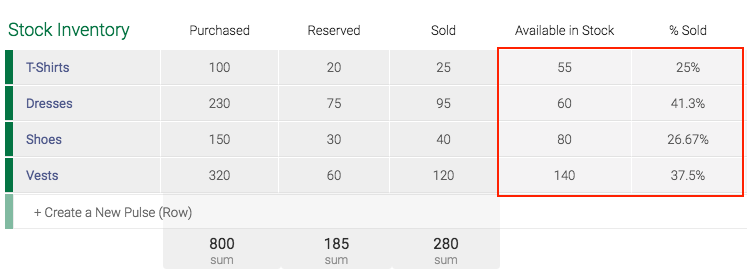This simple formula allows you to keep track of your shipments and easily find out if they are good to go in transit. There are a few options to calculate it but the most frequently used one is the following:Formula: (MULTIPLY((Height),(Length),(Width)))/6000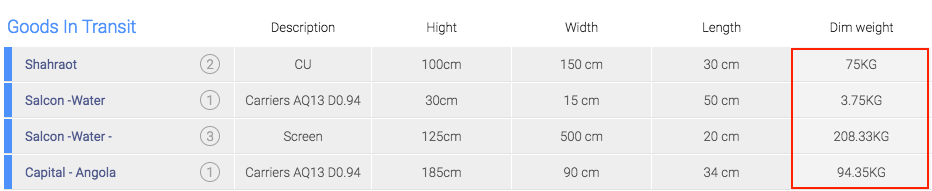# Use Cases using dates

Get the number of days between two datesFormula: DAYS({Due Date},{Start Date})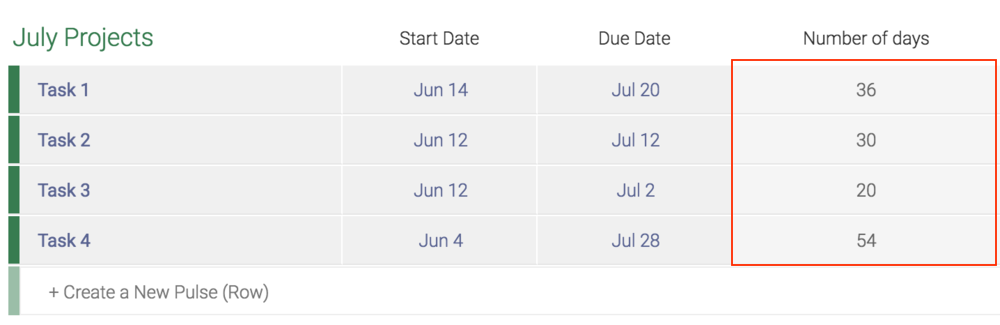Get numbers of days between two dates excluding the weekends

At monday.com, we are using a vacation board to keep track of everyone's holidays. We use the formula column to see how many working days each team member will be missing.Formula: DAYS({To},{From})-(2*(WEEKNUM({To})-WEEKNUM({From})))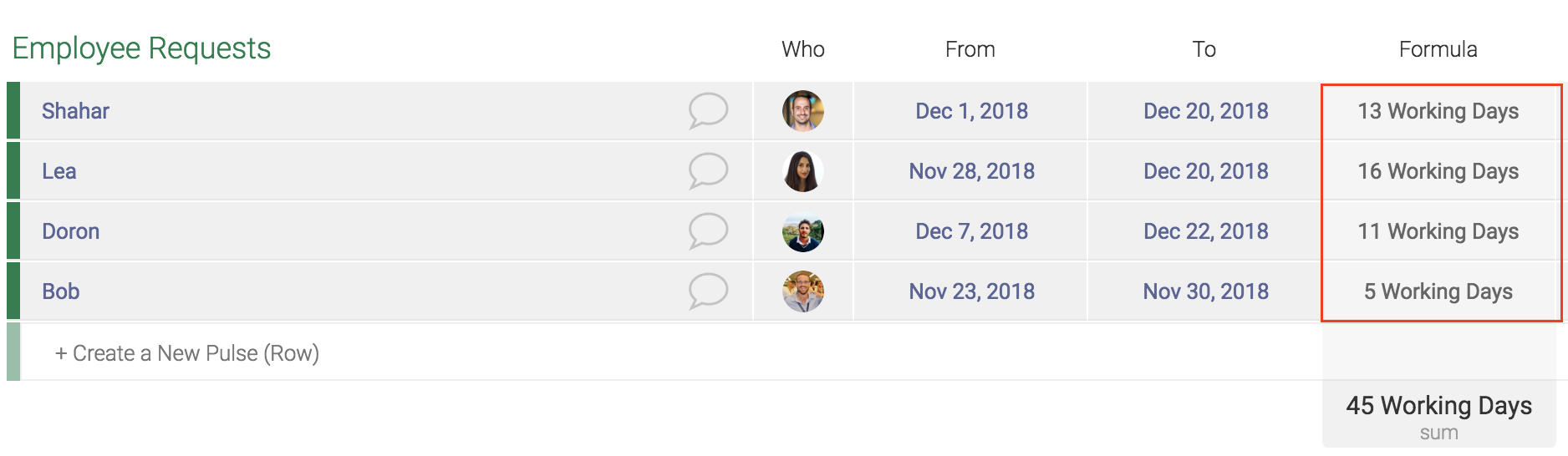Get the countdown between today and the due date of your project

I want to know how many days remain until the due date of my task.Formula: ROUND(DAYS({Due Date},TODAY()),0)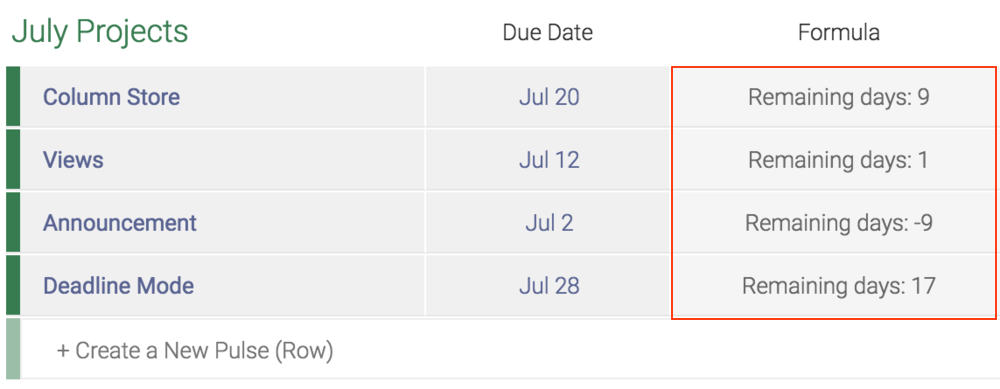In this example, we want to add 15 days to each date from the column "start date". This first formula will show you a result unformatted which might look a little clunky.Formula: (DATE(YEAR({Start Date}),MONTH({Start Date}),DAY({Start Date})+15))

This is why we recommend to use the below formula. The result is formatted and will look cleaner in your board.Formula: RIGHT(LEFT(CONCATENATE(DATE(YEAR({Start Date}),MONTH({Start Date}),DAY({Start Date})+15)),10),6)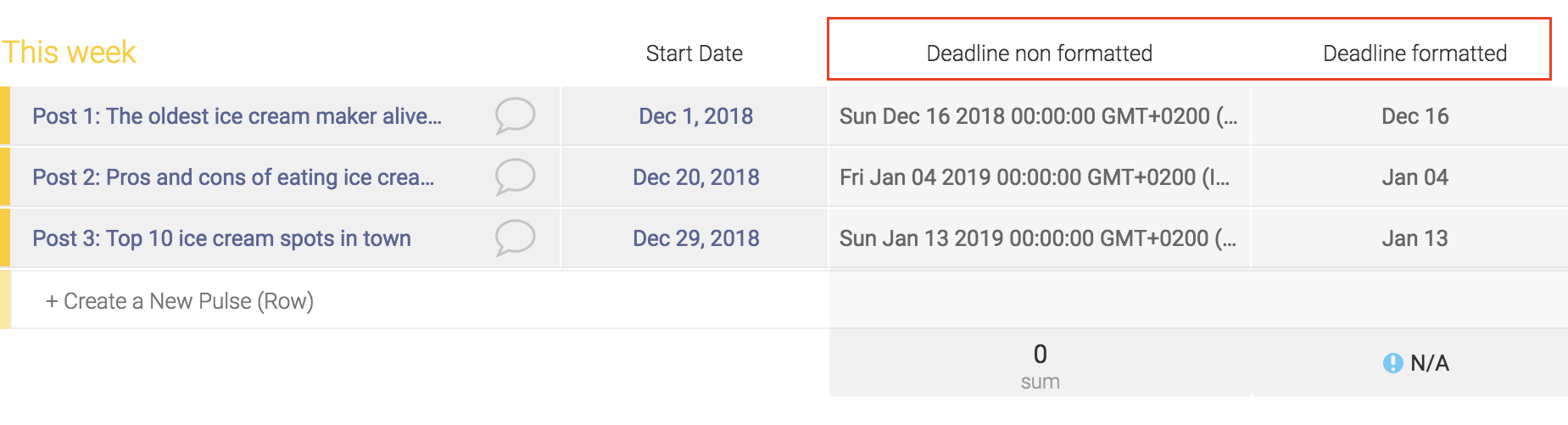# Some other useful use cases

In this example, I want to see if my bug is urgent or if it can wait. If the bug has been added more than 7 days ago, then it is urgent, if not, it can wait.Formula: IF(DAYS(TODAY(),{Creation Log})>7,"Urgent check now","Can wait")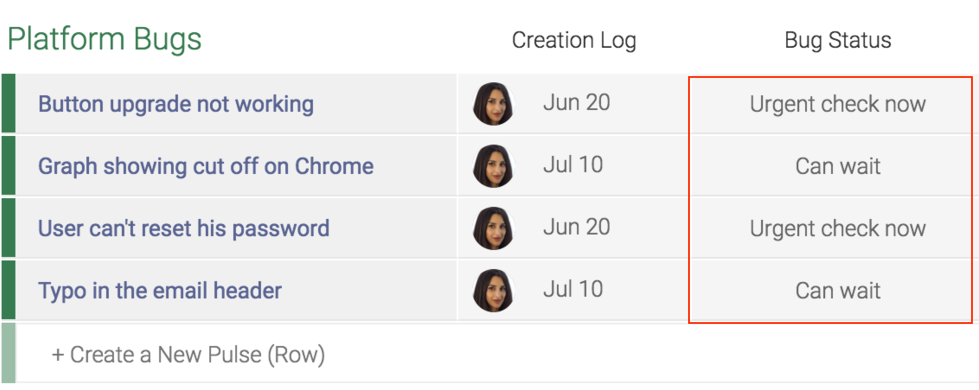Get a value based on several conditions

If the student got 80 or more at his test, the result is "Excellent". If the result is above 50, it is "good". If it is above 30, you"can do better". If the result is below 30, then it is "terrible".Formula: IF({Exam Score}>80,"Excellent",IF({Exam Score}>50,"Good",IF({Exam Score}>30,"Can do Better","Terrible")))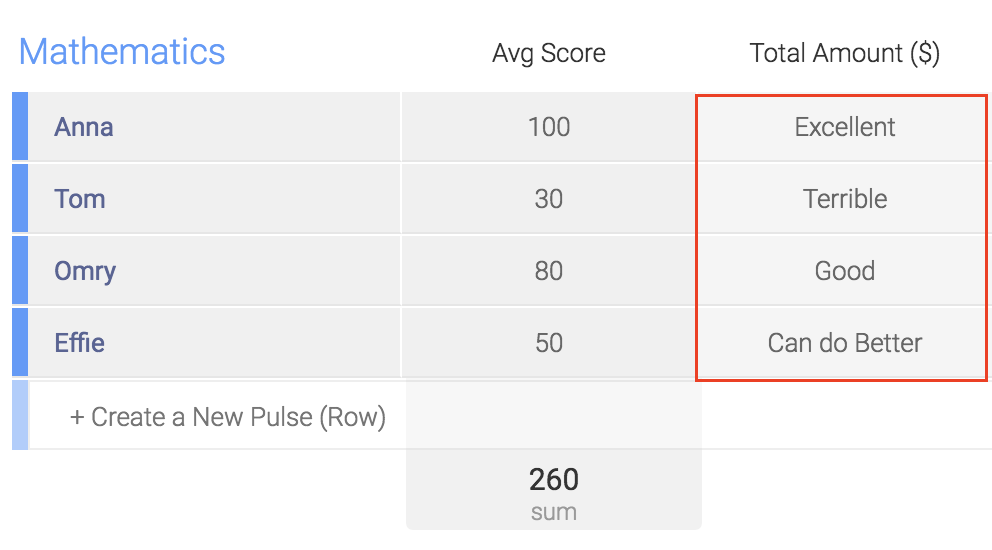Calculate a risk map for your investments

Create a risk map to estimate if an investment is high risk, medium risk or low risk. For that we need two data: the risk impact and the probability of the event happening. You can then add a status column to categorize each type of investment.Formula: MULTIPLY(({Risk Impact}*{Probability})/100)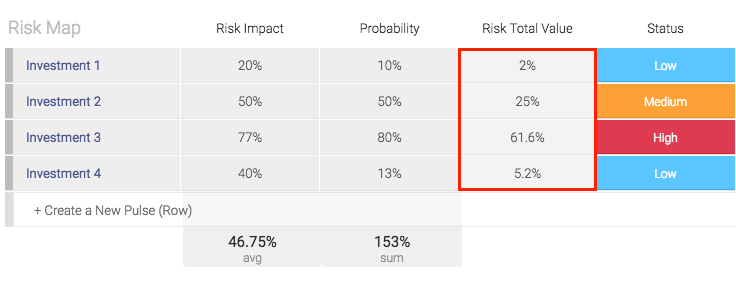Use Status labels to assign commission rates

In this example, let's say I manage a sales team with varying commission rates per project or salesperson.  You can use the labels from the status column to manipulate the formula to calculate different rates based on which label is selected:Formula: IF({Agent}="Agent 1",15,IF({Agent}="Agent 2",20,IF({Agent}="Agent 3",12)))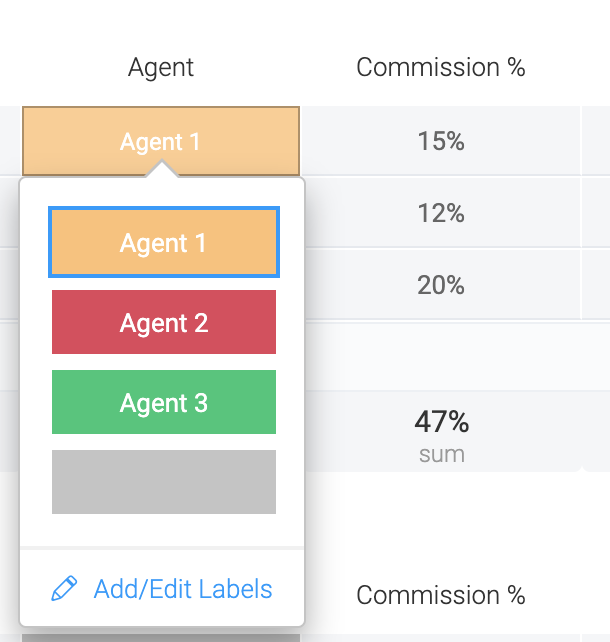Calculating how long somebody worked, subtracting their break

In this example, I'd like to calculate how long my employees have worked, minus their unpaid break time.  Using four "Hour" columns, I can create a formula to calculate just this:Formula: IF((HOURS_DIFF({Break End}, {Break Start})) > "0", (HOURS_DIFF((HOURS_DIFF({End}, {Start})), (HOURS_DIFF({Break End}, {Break Start})))), (HOURS_DIFF({End}, {Start})))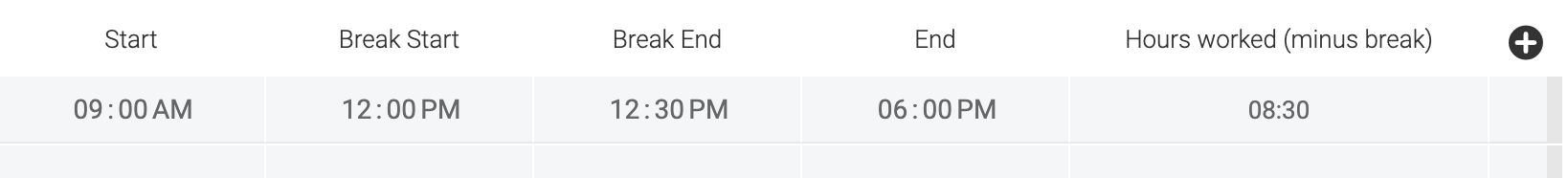If you have any further questions about the formula column please don't hesitate to reach out to our customer success team at [email protected]! We are here for you 24/7.